# Calculating a Missing Amount within Owner's Equity

The income statement for the calendar year 2022 will explain a portion of the change in the owner's equity between the balance sheets of December 31, 2021 and December 31, 2022. The other items that account for the change in owner's equity are the owner's investments into the sole proprietorship and the owner's draws (or withdrawals). A recap of these changes is the statement of changes in owner's equity. Here is a statement of changes in owner's equity for the year 2022 assuming that the Accounting Software Co. had only the eight transactions that we covered earlier.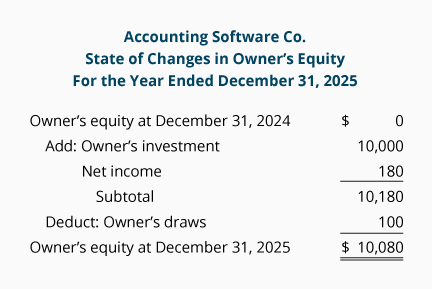### Example of Calculating a Missing Amount

The format of the statement of changes in owner's equity can be used to determine an unknown component. For example, if the net income for the year 2022 is unknown, but you know the amount of the draws and the beginning and ending balances of owner's equity, you can calculate the net income. (This might be necessary if a company does not have complete records of its revenues and expenses.) Let's demonstrate this by using some new hypothetical amounts: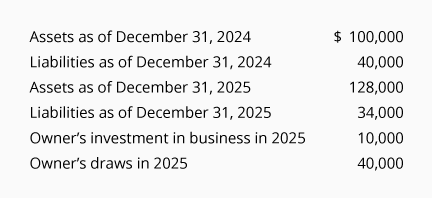Step 1.

The owner's equity at December 31, 2021 can be computed using the accounting equation: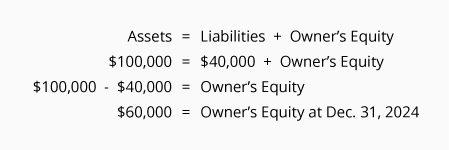Step 2.

The owner's equity at December 31, 2022 can be computed as well: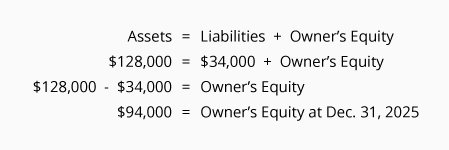Step 3.

Insert into the statement of changes in owner's equity the information that was given and the amounts calculated in Step 1 and Step 2: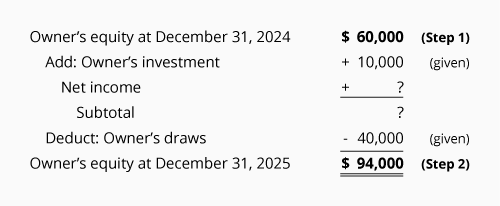Step 4.

The "Subtotal" can be calculated by adding the last two numbers on the statement: \$94,000 + \$40,000 = \$134,000. After this calculation we have: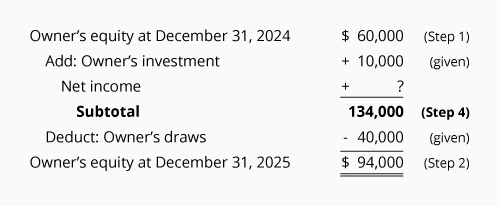Step 5.

Starting at the top of the statement we know that the owner's equity before the start of 2022 was \$60,000 and in 2022 the owner invested an additional \$10,000. As a result we have \$70,000 before considering the amount of Net Income. We also know that after the amount of Net Income is added, the Subtotal has to be \$134,000 (the Subtotal calculated in Step 4). The Net Income is the difference between \$70,000 and \$134,000. Net income must have been \$64,000.

Step 6.

Insert the previously missing amount (in this case it is the \$64,000 of net income) into the statement of changes in owner's equity and recheck the math: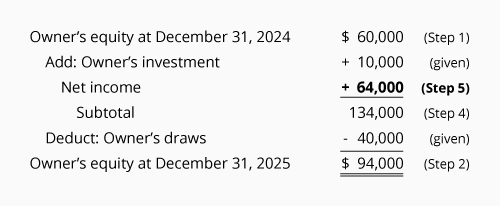Since the statement is mathematically correct, we are confident that the net income was \$64,000.

You can reinforce what you have learned by using our Quiz for the Accounting Equation and our Crossword Puzzle on the Accounting Equation.

The remaining parts of this topic will illustrate similar transactions and their effect on the accounting equation when the company is a corporation instead of a sole proprietorship.